Examples

Chapter 7 Class 11 Permutations and Combinations
Serial order wise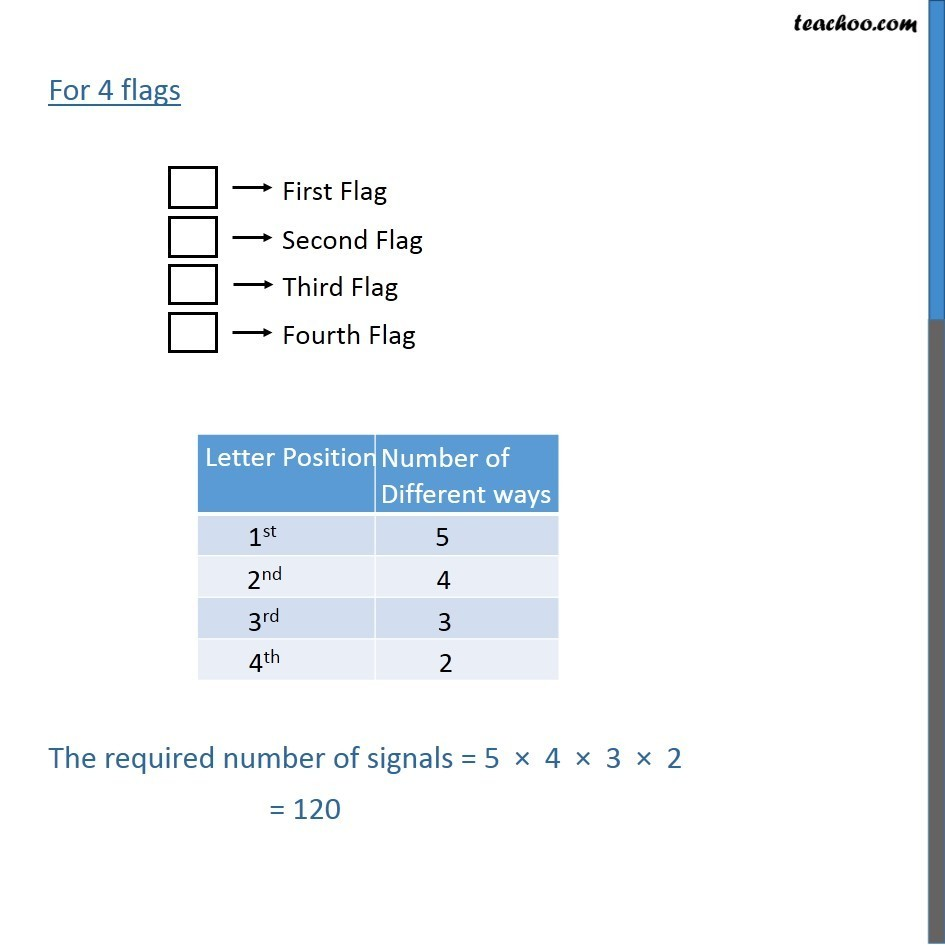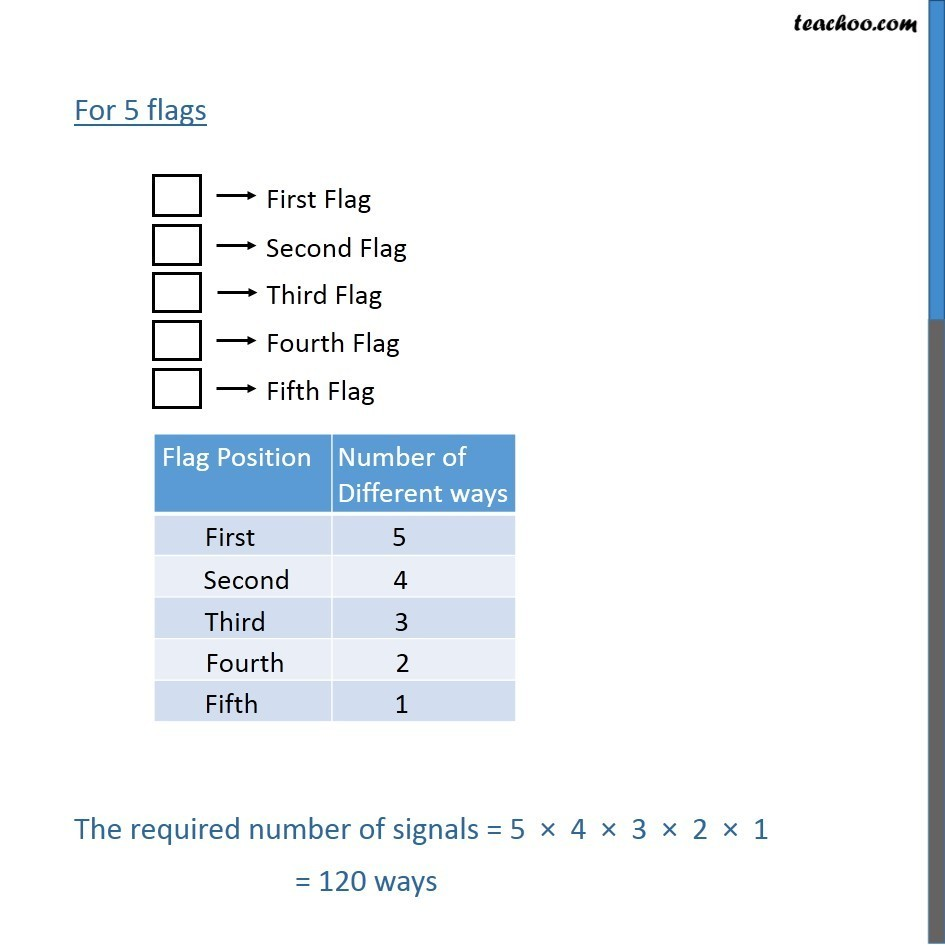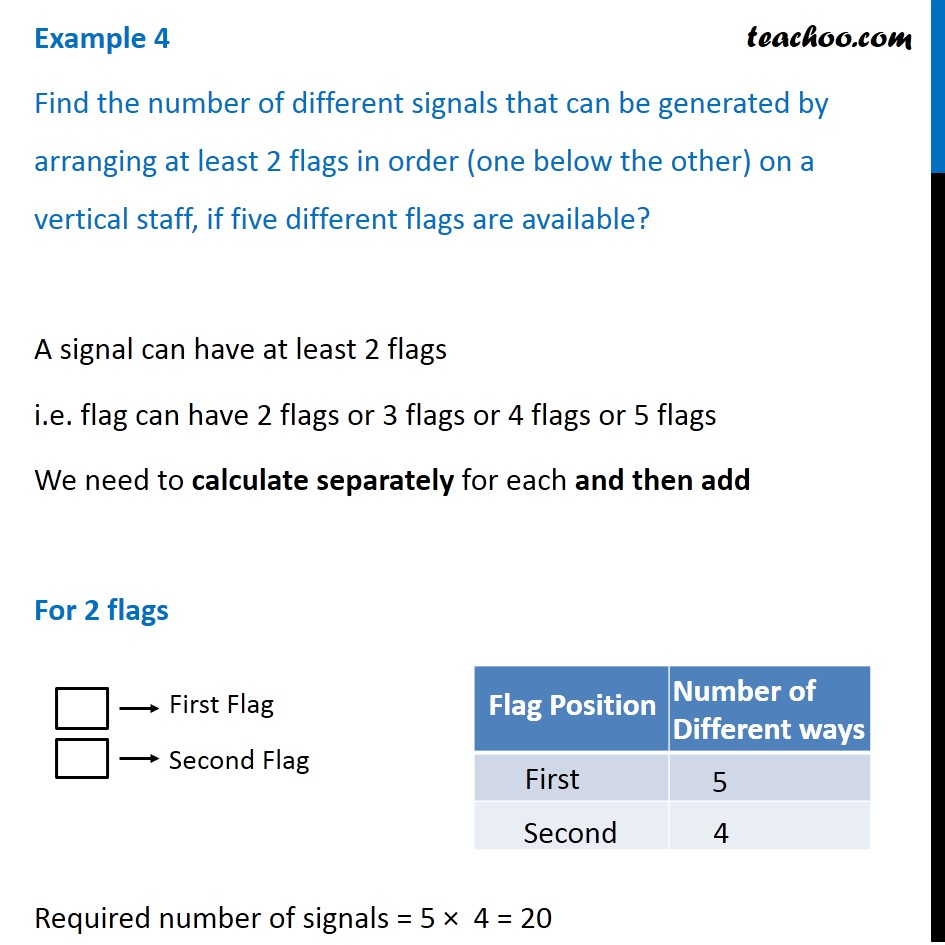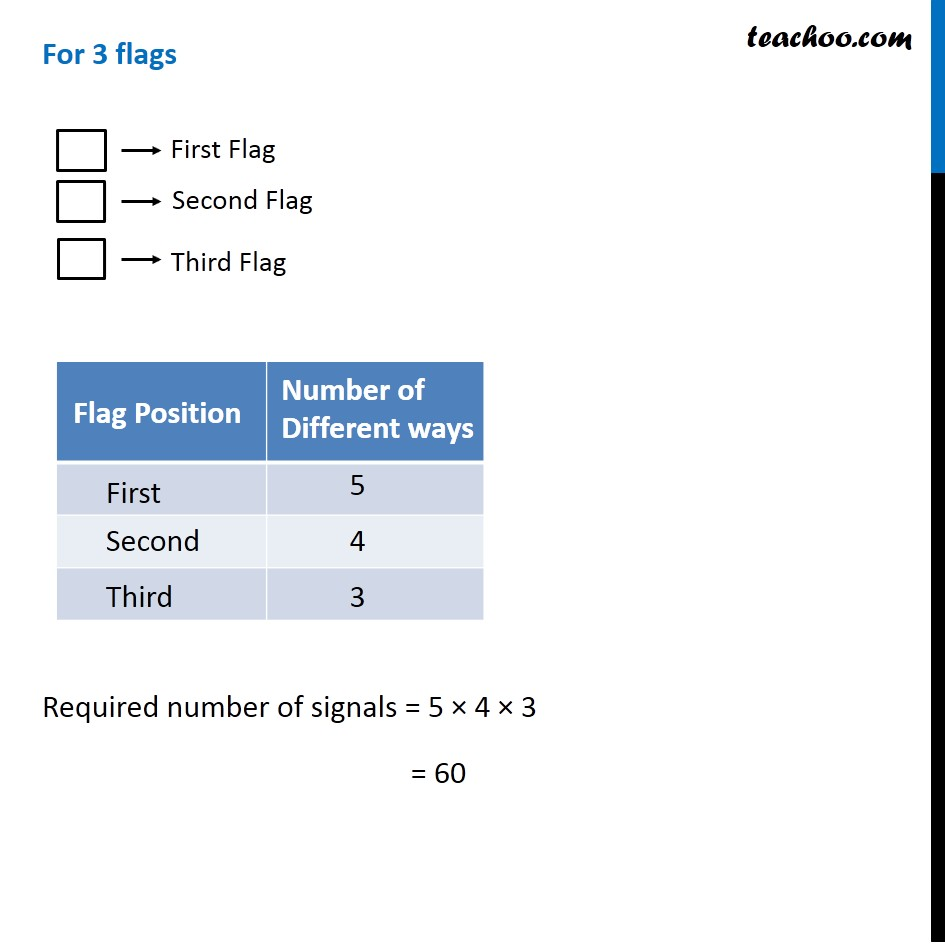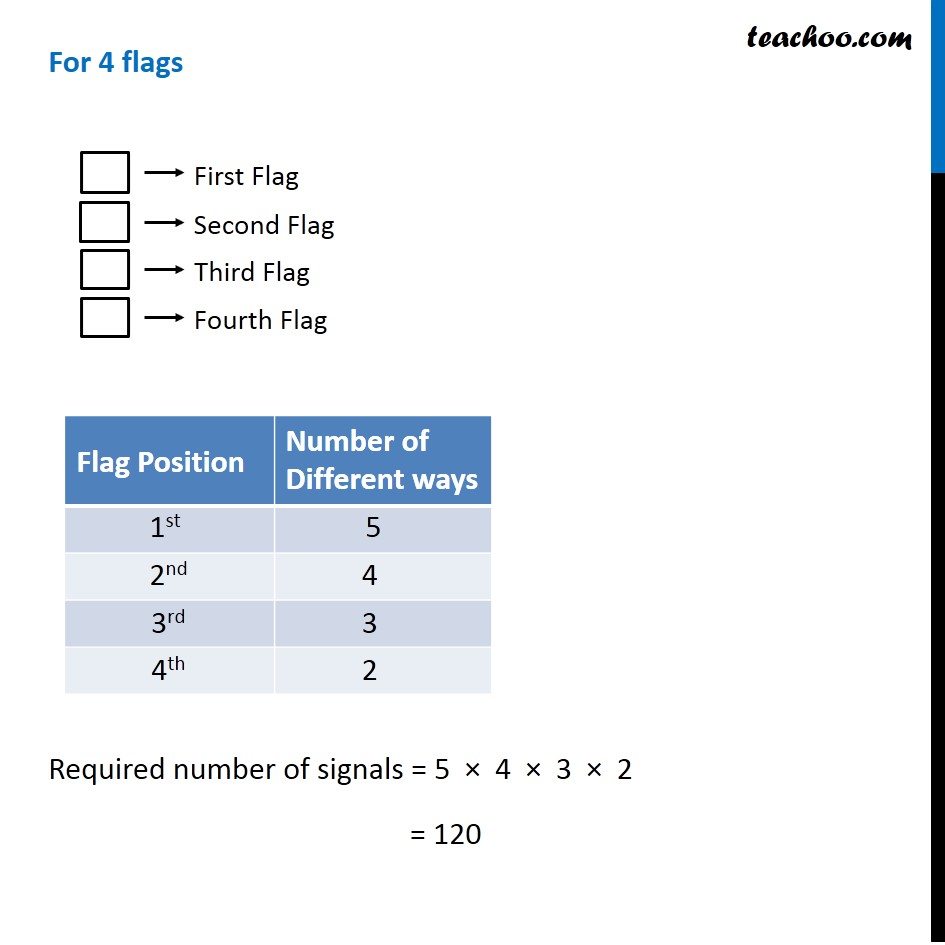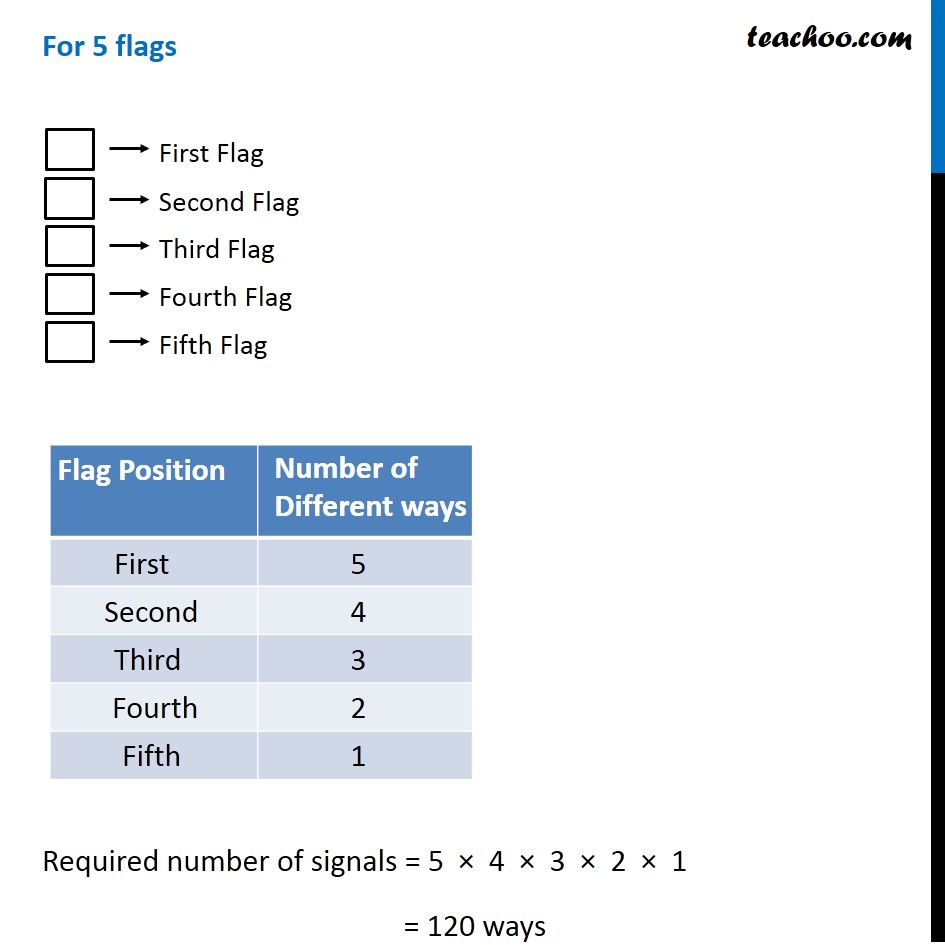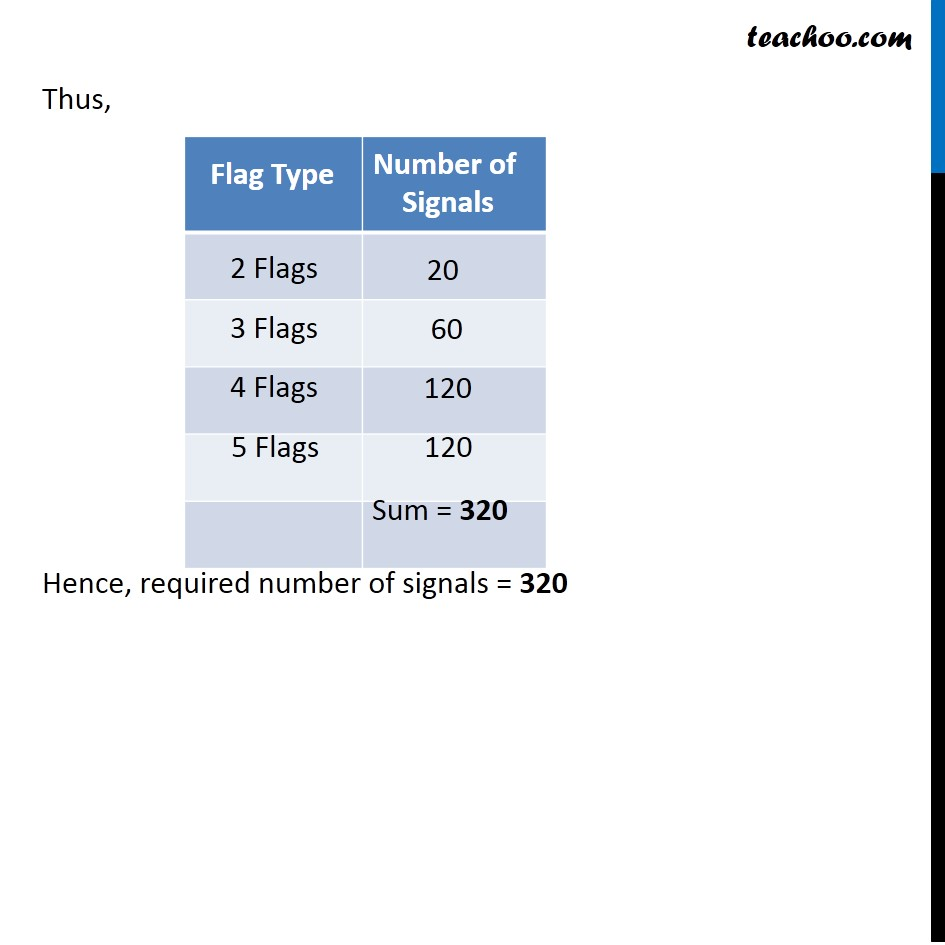Get live Maths 1-on-1 Classs - Class 6 to 12

### Transcript

Example 4 Find the number of different signals that can be generated by arranging at least 2 flags in order (one below the other) on a vertical staff, if five different flags are available? A signal can have at least 2 flags i.e. flag can have 2 flags or 3 flags or 4 flags or 5 flags We need to calculate separately for each and then add For 2 flags Required number of signals = 5 × 4 = 20 First Flag Second Flag First Flag For 3 flags Required number of signals = 5 × 4 × 3 = 60 For 4 flags Required number of signals = 5 × 4 × 3 × 2 = 120 First Flag Second Flag Fourth Flag For 5 flags Required number of signals = 5 × 4 × 3 × 2 × 1 = 120 ways Thus, Hence, required number of signals = 320